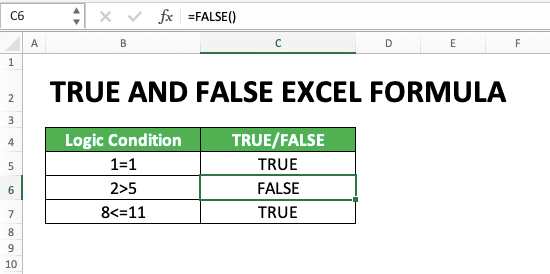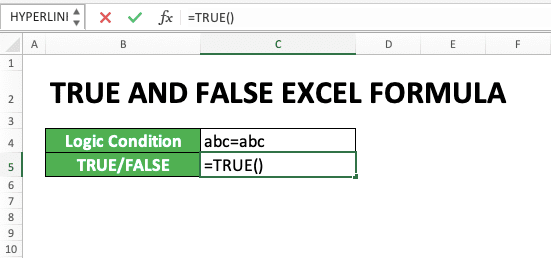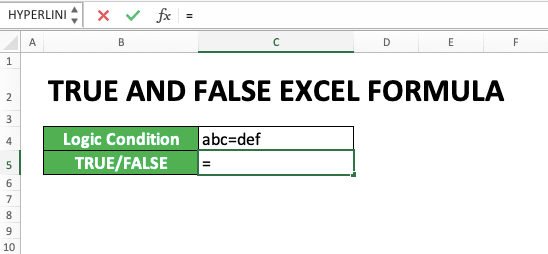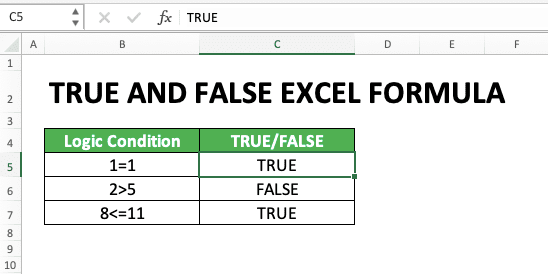How to Use TRUE and FALSE Formulas in Excel: Function, Example, and Writing - Compute Expert

# How to Use TRUE and FALSE Formulas in Excel: Function, Example, and Writing

Home >> Excel Tutorials from Compute Expert >> Excel Formulas List >> How to Use TRUE and FALSE Formulas in Excel: Function, Example, and Writing

In this tutorial, you will learn how to use TRUE and FALSE formulas in excel. These two formulas will produce logic values that you can use for the data processing that needs to use it.

Disclaimer: This post may contain affiliate links from which we earn commission from qualifying purchases/actions at no additional cost for you. Learn more

## TRUE and FALSE Formula Function in Excel

The usability/function of TRUE and FALSE formulas is to produce a TRUE or FALSE logic value in your cell/formula.

## Result

The result given by TRUE and FALSE formulas is a TRUE or FALSE logic value.

## Excel Version in Which TRUE and FALSE Formulas Can Start to Be Used

You can start to use these two formulas since excel 2003.

## The Way to Write Them and Their Input

Generally, the writing of TRUE and FALSE formulas in excel can be illustrated as follows.

TRUE:

=TRUE()

FALSE:

=FALSE()

Simple, isn’t it? These TRUE and FALSE formulas don’t need any input. Just type them like in the illustration above, enter, and your logic values will be produced.

=UPPER(text)

As you can see above, the input needed by this formula writing is just one. It is text, the data you want to change all its letters into uppercase letters.

## Example of Usage and Result

The following will give and explain the example of TRUE and FALSE formulas usage and result.

TRUE:FALSE:As you can see from the examples, the way to use TRUE and FALSE formulas is pretty easy.

You just need to type TRUE/FALSE, an open bracket sign, and a close bracket sign. These two formulas don’t need any input so you just need to press enter after you type them. The logic value based on the formula you type will then be given to you.

The same thing also applies if you use a TRUE/FALSE formula in other formulas. You just need to type TRUE() or FALSE() to use their results as the other formulas’ input.

## Writing Steps

The following will explain the steps to write TRUE and FALSE formulas. Each step will be explained with the help of an excel screenshot to make you learn it easier.

TRUE:

1. Type an equal sign ( = ) in the cell where you want to put your TRUE logic value2. Type TRUE (can be with large and small letters)3. Input an open bracket sign, followed by a close bracket sign4. Press enter
5. Done!FALSE:

1. Type an equal sign ( = ) in the cell where you want to put your TRUE logic value2. Type TRUE (can be with large and small letters)3. Input an open bracket sign, followed by a close bracket sign4. Press enter
5. Done!## Alternative 1: Write the Logic Value Directly

If you want, you can also get the same results as the TRUE and FALSE formulas through direct logic value typing. Type TRUE if you want a TRUE logic value and type FALSE if you want a FALSE logic value instead.

See the examples below.

TRUE:FALSE:Excel will automatically convert the TRUE/FALSE text you type into a logic value data type.

## Alternative 2: Use a Logic Condition

Want to get a logic value directly from a logic condition? You can do that also in excel.

Just type an equal sign before your logic condition in the cell where you want to put the logic value. Excel will automatically evaluate the logic condition and give a TRUE/FALSE result, depending on whether the condition is right/wrong.

In the logic condition you input in excel, you usually need an operator. You use the operator as a connector and evaluator between the first and second values in your logic condition. The often-used operators in excel and their meaning are as follows.

OperatorMeaning
=Equal to
<Less than
>More than
<=Less than or equal to
>=More than or equal to

The logic condition writing that can produce TRUE or FALSE in excel can be seen in the screenshot below.

TRUE:FALSE:Easy, isn’t it? You just need to type your logic condition in an excel formula format (with the equal sign in front of it). Press enter after you have done typing and the logic value from your logic condition evaluation will be produced.

## Exercise

After learning how to use TRUE and FALSE formulas in excel completely, now let’s do an exercise.

### Questions

Answer each question in the gray-colored cell based on the number!
1. Is the logic condition number 1 TRUE or FALSE? Use the TRUE/FALSE formula to answer it!
2. Is the logic condition number 2 TRUE or FALSE? Type a logic value text directly to answer it!
3. Is the logic condition number 3 TRUE or FALSE? Type the logic condition in a formula format to answer it!

TRUE and FALSE formulas are compatibility formulas made available by excel to interact with other spreadsheet applications. We usually never use them in a normal data processing.

Other formulas you need to learn too:

Get updated excel info from Compute Expert by registering your email. It's free!